## MP Board Class 9th Science Solutions Chapter 11 Work and Energy

### Work and Energy Intext Questions

Work and Energy Intext Questions Page No. 148

Question 1.
A force of 7 N acts on an object. The displacement is, say 8 m, in the direction of the force (Fig. below). Let us take it that the force acts on the object through the displacement. What is the work done in this case?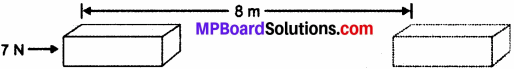The work done W on the body by the force is given by:
Work done = Force × Displacement
W = F × s
Given:
F = 7 N
s = 8 m
Hence, work done, W = 7 × 8
= 56 Nm
= 56 J.

Work and Energy Intext Questions Page No. 149

Question 1.
When do we say that work is done?
We can say a work is done whenever the conditions given below are satisfied:

1. A force is applied over the body.
2. A displacement of the body is caused by the applied force, along the direction of the applied force.

Question 2.
Write an expression for the work done when a force is acting on an object in the direction of its displacement.
When a force ‘F’ displaces a body by a distance ‘d’ in the direction of the applied force, then the work done ‘W’ on the body is given by:
Work done = Force × Displacement
W = F × d.Question 3.
Define 1 J of work.
1 J is the amount of work done when an object is provided with a force of 1 N that displaces it through a distance of 1 m in the direction of the applied force.

Question 4.
A pair of bullocks exerts a force of 140 N on a plough. The field being ploughed is 15 m long. How much work is done in ploughing the length of the field?
Here,
Applied force, F = 140 N
Displacement, d = 15 m
We know,
Work done is given by the expression:
Work done = Force × Displacement
W = F × d
So,
W= 140 × 15 = 2100 J
Hence, 2100 J of work is done in ploughing the length of the field.

Work and Energy Intext Questions Page No. 152

Question 1.
What is the kinetic energy of an object?
The energy attained by or generated in a body due to its action or motion is called kinetic energy. Every object which possesses motion contain a kinetic energy. A body uses kinetic energy to do work. Kinetic energy can be used for any work to be performed. Kinetic energy is useful to generate other forms of energy too. It is expressed by KE and can be calculated by the following formula:
Ek = $$\frac { 1 }{ 2 }$$ mv2
Here, m represents mass of object.
And ‘V’ gives the velocity by which object is shifting or working.Question 2.
Write an expression for the kinetic energy of an object.
Energy Ek is proportional to:

• Object’s mass and
• Square of its velocity.

Energy Ek due to a moving object with a body mass ‘m’ which is moving with a velocity v, can be given by the expression,
Ek = $$\frac { 1 }{ 2 }$$ mv2
Its S.I. unit is joule (J).

Question 3.
The kinetic energy of an object of mass, m moving with a velocity of 5 ms-1 is 25 J. What will be its kinetic energy when its velocity is doubled? What will be its kinetic energy when its velocity is increased three times?
Given:
K.E. of the object = 25 J
Velocity of the object, v = 5 m/s
Putting value to formula:
∵ K.E = $$\frac { 1 }{ 2 }$$ mv2

• m = 2 × K.E / v2
• m = 2 × $$\frac { 25 }{ 25 }$$ = 2 kg

Condition 1:
If velocity is double, v = 2 × 5 = 10 m/s
∴ K.E. (for v = 10 m/s) = $$\frac { 1 }{ 2 }$$ mv2 = $$\frac { 1 }{ 2 }$$ × 2 × 100 = 100 J

Condition 2:
If velocity is tripled, v = 3 × 5 = 15 m/s
∴ K.E. (for v = 10 m/s) = $$\frac { 1 }{ 2 }$$ mv2 = $$\frac { 1 }{ 2 }$$ × 2 × 225 = 225 J.

Work and Energy Intext Questions Page No. 156

Question 1.
What is Power?
Work done is calculated by the amount of power consumption. Power can be understood by the term efficiency of an object to consume or generate energy. So, power is the rate of doing work or the rate of transfer of energy. If W is the amount of work done in time t, then power is given by the expression,
Power = $$\frac { Work }{ Time }$$ = $$\frac { Energy }{ Time }$$
or
P = $$\frac { W }{ T }$$
It is calculated in watt (W).

Question 2.
Define 1 watt of power.
As we know that:
Power = $$\frac { Work }{ Time }$$
Hence,
A body is said to have power of 1 watt if its work is equal to 1 joule in 1 s, i.e.,
1 W = $$\frac { 1J }{ 1s }$$Question 3.
A lamp consumes 1000 J of electrical energy in 10 s. What is its power?
Power = $$\frac { Work }{ Time }$$
Given,
Work done = Energy consumed by the lamp = 1000 J
Time = 10 s
Putting values,
Power = $$\frac { 1000 }{ 10 }$$ = 100 Js-1
= 100 W.

Question 4.
Define average power.
When efficiency of an operator is changed with time, average power is calculated.
The average power of an object is defined as the total work done by it in the total time taken.
Total time taken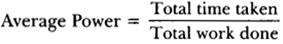### Work and Energy NCERT Textbook Exercises

Question 1.
Look at the activities listed below. Reason out whether or not work is done in the light of your understanding of the term ‘work’.

• Suma is swimming in a pond.
• A donkey is carrying a load on its back.
• A wind – mill is lifting water from a well.
• A green plant is carrying out photosynthesis.
• An engine is pulling a train.
• Food grains are getting dried in the sun.
• A sailboat is moving due to wind energy.

Work is done whenever the two given conditions are satisfied:

• A force is applied over the body.
• A displacement of the body is caused by the applied force, along the direction of the applied force.

Hence, work is done in case:

• Suma is swimming in a pond.
• A wind – mill is lifting water from a well.
• An engine is pulling a train.
• A sailboat is moving due to wind energy.

Work is not being done in case:

• A donkey is carrying a load on its back.
• A green plant is carrying out photosynthesis.
• Food grains are getting dried in the sun.

Explanation:

1. Suma applies a force to push the water backwards which causes a displacement. Hence, work is done by Suma while swimming.
2. While carrying a load, the donkey has to apply a force in the upward direction. But, displacement is exchanged with shifting, so the work done is zero.
3. A wind – mill works against the gravitational force to lift water. Hence, work is done.
4. In this case, chemical change occurs not a physical. Therefore, the work done is zero.
5. An engine applies force to pull the train. Therefore, there is a displacement in the train in the same direction. Hence, work is done by the engine on the train.
6. This is an example of evaporation. Hence, the work done is zero.
7. Wind energy applies a force on the sailboat to push it in the forward direction. Therefore, there is a displacement in the boat in the direction of force. Hence, work is done by wind on the boat.

Question 2.
An object thrown at a certain angle to the ground moves in a curved path and falls back to the ground. The initial and the final points of the path of the object lie on the same horizontal line. What is the work done by the force of gravity on the object?
Gravitational forces are proportional to ‘h’ which is vertical displacement and work done by the force of gravity is considered only if vertical displacement occurs. Vertical displacement is given by the difference in the initial and final positions / heights of the object which is zero. In this case work done by gravity is given by thy expression,
W = mgh
Where,
h = Vertical displacement = 0
W = mg × 0 = 0 J
Therefore, the work done by gravity on the given object is zero joule.Question 3.
A battery lights a bulb. Describe the energy changes involved in the process.
When a battery lights a bulb, then the chemical energy of the battery is converted into electrical energy. When the bulb receives this electrical energy, then it converts it into light and heat energy. Hence, the transformation of energy is as follows:
Chemical Energy → Electrical Energy → Light Energy + Heat Energy

Question 4.
Certain force acting on a 20 kg mass changes its velocity from 5 ms-1 to 2 ms-1. Calculate the work done by the force.
Kinetic energy is given by the expression, (Ek) = $$\frac { 1 }{ 2 }$$ mv2.
Where,
Ek = Kinetic energy of the object moving with a velocity,
v Kinetic energy when the object was moving with a velocity 5 ms-1.
(Ek)5 = $$\frac { 1 }{ 2 }$$ × 20 × (5)2 = 250 J
Kinetic energy when the object was moving with a velocity 2 ms-1.
(Ek)2 = $$\frac { 1 }{ 2 }$$ × 20 × (2)2 = 40 J

Question 5.
A mass of 10 kg is at a point A on a table. It is moved to a point B. If the line joining A and B is horizontal, what is the work done on the object by the gravitational force? Explain your answer.
Work done by gravity depends only on the vertical displacement of the body. It does not depend upon the path of the body.
Therefore, by the expression,
W = mgh
Here,
Vertical displacement, h = 0
W = mg × 0 = 0
Hence, the work done by gravity on the body is zero.

Question 6.
The potential energy of a freely falling object decreases progressively. Does this violate the law of conservation of energy? Why?
No. In freely falling object only potential energy decreases progressively, but at same time kinetic energy increases and total of both remains equal to initial energy.
Total energy = Potential energy + Kinetic energy
So, this process does not violate the law of conservation of energy. During the process, total mechanical energy of the body remains equal.

Question 7.
What are the various energy transformations that occur when you are riding a bicycle?
When we ride a bicycle, the chemical energy of muscles of rider’s body gets transferred into heat energy and kinetic energy of the bicycle. Heat energy is changed to physical energy. Kinetic energy provides a velocity to the bicycle. The transformation can be shown as:
Muscular energy → Kinetic energy + Heat energy
During the transformation, the total energy remains conserved.Question 8.
Does the transfer of energy take place when you push a huge rock with all your might and fail to move it? Where is the energy you spend going?
When we push a huge rock, there is no transfer of muscular energy to the stationary rock. Here, the energy is completely spent doing work (pushing) against friction between the ground and the rock.

Question 9.
A certain, household has consumed 250 units of energy during a month. How much energy is this in joules?
1 unit of energy is equal to 1 kilowatt hour (kWh).
1 unit = 1 kWh
1 k Wh = 3.6 × 106 J
Therefore, 250 units of energy
= 250 × 3.6 × 106 = 9 × 108 J.

Question 10.
An object of mass 40 kg is raised to a height of 5 m above the ground. What is its potential energy? If the object is allowed to fell, find its kinetic energy when it is half – way down.
Gravitational potential energy is given by the expression,
W = mgh
Where,
h = Vertical displacement = 5 m
m = Mass of the object = 40 kg
g = Acceleration due to gravity = 9.8 ms-2
∴ W = 40 × 5 × 9.8 = 1960 J.
At half – way down, the potential energy of the object will be $$\frac { 1960 }{ 2 }$$ = 980 J.
At this point, the object has an equal amount of potential and kinetic energy.
This is due to the law of conservation of energy.
Hence, half – way down, the kinetic energy of the object will be 980 J.Question 11.
What is the work done by the force of gravity on a satellite moving round the earth? Justify your answer.
Work is done whenever the two given conditions are satisfied:

1. A force acts on the body.
2. There is a displacement of the body by the application of force in or opposite to the direction of force.

If the direction of force is perpendicular to displacement, then the work done is zero. When a satellite moves around the Earth, then the direction of force of gravity on the satellite is perpendicular to its displacement. Hence, the work done on the satellite by the Earth is zero.

Question 12.
Can there be displacement of an object in the absence of any force acting on it? Think. Discuss this question with your friends and teacher.
Yes. For a uniformly moving object, suppose an object is moving with constant velocity. The net force acting on it is zero. But, there is a displacement along the motion of the object. Hence, there can be a displacement without a force.

Question 13.
A person holds a bundle of hay over his head for 30 minutes and gets tired. Has he done some work or not? Justify your answer.
When a person holds a bundle of hay over his head, then there is no displacement in the bundle of hay. And since
displacement of the body by the application of force is required to prove that work is done, no work is done here.
Here, force of gravity is acting on the bundle, but the person 1 is not applying any force on it. Hence, in the absence of force, work done by the person on the bundle is zero.

Question 14.
An electric heater is rated 1500 W. How much energy does it use in 10 hours?
Energy consumed by an electric heater can be obtained with the help of the expression,
P = $$\frac { W }{ t }$$
Where,
Power rating of the heater, P = 1500, W = 1.5 kW
Time for which the heater has operated, t = 10 h
Work done = Energy consumed by the heater
Therefore,
Energy consumed = Power × Time = 1.5 × 10 = 15 kWh
Hence, the energy consumed by the heater in 10 h is 15 kWh.Question 15.
Illustrate the law of conservation of energy by discussing the energy changes which occur when we draw a pendulum bob to one side and allow it to oscillate. Why does the bob eventually come to rest? What happens to its energy eventually? Is it a violation of the law of conservation of energy?
According to law of conservation of energy, energy can be neither created nor destroyed. It can only be converted from one form to another. Considering the case of an oscillating pendulum.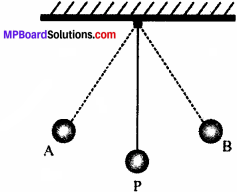When a pendulum moves from its actual position P to either of its extreme point A or B, it rises through a height h above the mean level P. Here at this point, the kinetic energy of the bob changes into potential energy and the kinetic energy becomes zero, and the bob possesses only potential energy.

When it moves towards point P, its potential energy decreases progressively. And the kinetic energy increases. As the bob reaches point P, its potential energy becomes zero and the bob possesses only kinetic energy. This process is repeated as long as the pendulum oscillates.

The pendulum loses its kinetic energy to overcome atmospheric friction and stops after some time. Hence law of conservation of energy is not violated and the total energy of the pendulum and the surrounding system remain conserved.

Question 16.
An object of mass, m is moving with a constant velocity, v. How much work should be done on the object in order to bring the object to rest?
We know that Kinetic energy of an object of mass m, moving with a velocity, v is given by:
Ek = $$\frac { 1 }{ 2 }$$ mv2
To bring the object to rest, total energy must be consumed.
So, $$\frac { 1 }{ 2 }$$ mv2 amount of work is required to be done on the object.

Question 17.
Calculate the work required to be done to stop a car of 1500 kg moving at a velocity of 60 km/h?
Kinetic energy, Ek = $$\frac { 1 }{ 2 }$$ mv2
Where,
Mass of car, m = 1500 kg
Velocity of car, v = 60 km/h
= 60 × $$\frac { 5 }{ 18 }$$ ms-1
Ek = $$\frac { 1 }{ 2 }$$ × 1500 × [60 × $$\frac { 5 }{ 18 }$$]2
= 20.8 × 104 J.
Hence, 20.8 × 104 J of work is required to stop the car.

Question 18.
In each of the following a force, F is acting on an object of mass, m. The direction of displacement is from west to east shown by the longer arrow. Observe the diagrams carefully and state whether the work done by the force is negative, positive or zero.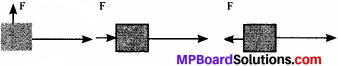Case I: Here, the direction of force acting on the block is perpendicular to the displacement. Therefore, work done will be zero.

Case II: Here, the direction of force acting on the block is in the direction of displacement. Therefore, work done will be positive.

Case III: Here, the direction of force acting on the block is opposite to the direction of displacement. Therefore, work done will be negative.Question 19.
Soni says that the acceleration in an object could be zero even when several forces are acting on it. Do you agree with her? Why?
When all the forces cancel out each other, acceleration in an object will be zero even when several forces are acting on it. And as for a uniformly moving object, the net force acting on the object is zero. Hence, the acceleration of the object is zero. Hence, Soni is right.

Question 20.
Find the energy in kWh consumed in 10 hours by four devices of power 500 W each.
We know,
P = $$\frac { W }{ t }$$
Given,
Power of the device (P) = 500 W = 0.50 kW
Total Time (t) = 10 h
Since,
Work done = Energy consumed by the device
Therefore, energy consumed
= Power × Time = 0.50 × 10 = 5 kWh
Hence, the energy consumed by four equal rating devices in 10 h will be:
4 × 5 kWh = 20 kWh
= 20 Units.

Question 21.
A freely falling object eventually stops on reaching the ground. What happens to its kinetic energy?
As the object hits the ground, its kinetic energy gets converted into heat energy and sound energy. Sometimes, it also deform the ground and itself depending upon the nature of the ground and the amount of kinetic energy of the object. Freely falling object towards the ground feels following changes:

• Its potential energy decreases and kinetic energy increases.
• When the object touches the ground, all its potential energy gets converted into kinetic energy.

### Work and Energy Additional Questions

Work and Energy Multiple Choice Questions

Question 1.
S.I. unit of work is ____________ .
(a) Newton
(b) Joule
(c) Watt
(d) All.
(b) Joule

Question 2.
Work done is applied and displacement occurred is acute ____________ .
(a) Negative
(b) Zero
(c) Positive
(d) None.
(c) Positive

Question 3.
Work done is negative, if angle formed between force applied and displacement occurred is ____________ .
(a) Obtuse
(b) Right angle
(c) Acute
(d) Zero.
(a) Obtuse

Question 4.
Work is done if displacement of object occurs in ____________ .
(a) Opposite the direction of force
(b) The direction of force
(c) Zero
(d) Infinite.
(b) The direction of forceQuestion 5.
Force is to work done ____________ .
(a) Inverse
(b) Zero
(c) Proportional
(d) Infinite.
(c) Proportional

Question 6.
If direction of applied force and displacement are perpendicular then resultant work will be ____________ .
(a) Zero
(b) Positive
(c) Negative
(d) Infinite.
(a) Zero

Question 7.
Writing for two hours is equal to work ____________ .
(a) 1 joule
(b) 2 joule
(c) Zero
(d) None.
(c) Zero

Question 8.
Falling ball will have ____________ .
(a) Kinetic energy
(b) Potential energy
(c) Both
(d) None.
(c) BothQuestion 9.
If mass of an object is doubled over a pully, force required to displacement upto previous destination will be ____________ .
(a) Double
(b) Half
(c) Four times
(d) Equal.
(a) Double

Question 10.
Change in kinetic energy, if velocity of an object is doubled will be ____________ .
(a) Double
(b) Half
(c) Equal
(d) 4 times.
(d) 4 times.

Question 11.
Rate at which work is done is called ____________ .
(a) Work
(b) Power
(c) K.E.
(d) P.E.
(b) Power

Question 12.
Running wings of a fan shows an example of ____________ .
(a) K.E.
(b) P.E.
(c) Work
(d) Power.
(a) K.E.

Work and Energy Very Short Answer Type Questions

Question 1.
Give a formula / expression to give total energy.
Total energy = Potential energy + Kinetic energy.

Question 2.
How displacement is related to work?
Displacement is proportionally related to work.Question 3.
What is a positive work?
When displacement occurs in direction of force applied and object shifted forms an acute angle with force, work is termed as positive.

Question 4.
What kind of force is applied in a lift?
Upward and gravitational force is applied in a lift.

Question 5.
What kind of quantity is power?
It is scalar.

Question 6.
What will be the potential energy of an object at ground?
PE = mgh
Here, h = 0
So, PE = mg.

Question 7.
What will be the work done if no force is being applied?
Since, W = F × S
If F = 0;
W = 0 × S
Hence, W = 0
∴ No work will be done.

Question 8.
Name three forms of energy.
Potential energy, Kinetic energy and Gravitational energy.

Question 9.
Write expression to calculate kinetic energy if mass of an object is m and its velocity is v.
K.E. = $$\frac { 1 }{ 2 }$$ mv2.

Question 10.
Write expression for potential energy for an object being shifted to ‘d’ height and ‘a’ mass.
PE = agd.

Question 11.
If a machine consumes 1000 J of energy in a second and runs for 5 hours, then calculate total energy consumed.
P = $$\frac { W }{ t }$$
= 1000 × (5 × 3600)
= 18000000 joule
or
= 1.8 × 107 joule.Question 12.
What happen to potential and kinetic energy of a falling object?
P.E. = decrease to zero
K.E. = increase from zero
Total P.E. converts to K.E.

Work and Energy Short Answer Type Questions

Question 1.
If a pulley is pulling a box towards itself with a force equal to 10 N and have drawn the box upto 2 m. Calculate the work done by it.
Given, F = 10 N and s = 2 m
We know W = F × s
Putting values = 10 × 2
W = 20 Nm
or
20 J.

Question 2.
If a hammer operates over a pully machine with 21 J and pulls the balls to 7 m, Calculate the force applied by the hammer.
Given:
W = 21 J and s = 7 m
Using formula W = $$\frac { F }{ s }$$
or
F = $$\frac { W }{ s }$$
Putting values F = $$\frac { 21 }{ 7 }$$ = 3 N
Hence, F = 3 N was applied by the hammer.

Question 3.
Calculate the work done by a pair of bullocks if an object is pulled by them for 10 m which has mass equal to 20 kg and an acceleration of 20 ms-1.
Given, m = 20 kg, a = 20 ms-1, s = 10 m
Using formula W = F × s
or
W = m × a × s
Putting values = 20 × 20 × 10
= 4000 kg ms-2 m = 4000 J.

Question 4.
Give two examples of energy produced due to work or action.
Examples showing production of kinetic energy:

1. Heat energy in body after exercise.
2. Generation of kinetic energy in a ball after falling through a point.

Question 5.
Read the following examples and tell in which case work is being done?

1. Manish is riding bicycle in a circular path.
2. John throws a pebble which comes back to initial height of ground.
3. Reading books for 2 hours.
4. Cooking pizza.

Work is being done in none case because:

1. Case 1: In a circular path displacement is zero, hence work done is zero.
2. Case 2: Again no displacement occurs.
3. Case 3: Any kind of force is not being used, hence zero work.
4. Case 4: No force is applied here, so zero work.Question 6.
(a) Under what conditions work is said to be done?
(b) A porter lifts a luggage of 1.5 kg from the ground and puts it on his head 1.5 m above the ground. Calculate the work done by him on the luggage.
(a) Conditions for work to be done:

1. Force should be applied.
2. Body should move in the line of action of force.
3. Angle between force and displacement should not be 90°.

(b) Given,
Mass of luggage, m = 15 kg
Displacement, s = 1.5 m
Using formula:
Work done i.e., W = F × s = mg × s
= 15 × 10 × 1.5 = 225 J.

Question 7.
Four persons jointly lift a 250 kg box to a height of 1 m and hold it.

1. Calculate the work done by the persons in lifting the box.
2. How much work is done for just holding the box?
3. Why do they get tired while holding it? (g = 10 ms2)

1. Given, F = 250 × 10 = 2500 N
s = 1 m
W = F × s = 2500 × 1 = 2500 J.
2. Zero work, as there is no displacement.
3. Men are applying a force which is opposite and equal to the gravitational force acting on the box. Muscular effort is involved and therefore persons feel tried.

Question 8.
(i) Justify that “a body at a greater height has larger energy”.
(ii) A body of mass 2 kg is thrown up at a velocity of 10 m/s. Find the potential energy at the highest point.
(i) When an object is placed at a greater height, the height increases from the reference level (Velocity remains constant i.e., zero). Hence by comparing the potential energy at two points, we can see that the P.E. at greater height will be larger.

(ii) Given,
Using formula m = 2 kg, v = 10 m/s
Initial KE = $$\frac { 1 }{ 2 }$$ mv2
= $$\frac { 1 }{ 2 }$$ × 2 × (10)2 = 100 J
Height will be
h = $$\frac { { v }^{ 2 }-{ u }^{ 2 } }{ 2g }$$ = $$\frac { { 0 }^{ 2 }-{ 10 }^{ 2 } }{ 2×10 }$$
= $$\frac { -100 }{ 20 }$$ = -5 m
So, magnitude of height = 5 m and
P.E. at highest point = mgh
= 2 × 10 × 5
= 100 J.

Question 9.
A light and a heavy object have the same momentum. What is the ratio of their kinetic energies? Which one has a larger kinetic energy?
p1 = m1v1, p2 = m2v2
But p1= p2  or  m1v1 = m2v2
and If m1 < m2 then v1 > v2
(K.E.)1 = $$\frac { 1 }{ 2 }$$ m1v12
(K.E.)2 = $$\frac { 1 }{ 2 }$$ m2v22
(K.E.)1 = $$\frac { 1 }{ 2 }$$ (m1v1)v1 = $$\frac { 1 }{ 2 }$$ p1v1
(K.E.)2 = $$\frac { 1 }{ 2 }$$ (m2v2)v2 = $$\frac { 1 }{ 2 }$$ p2v2.

Question 10.
(i) Define 1 kWh. Relate it to joules.
(ii) Find the energy in kWh in the month of September by four devices of power 100 W each, if each one of them is used for 10 hours daily.
(i) 1 kWh is the energy used in 1 hour at the rate of 1000 J/s (or 1 kW)
1 kWh = 3.6 × 106 J

(ii) Energy consumed by four devices in the month of September
= 4 × $$\frac { 100 }{ 1000 }$$ × 10 × 30
= 120 kWh.

Work and Energy Long Answer Type Questions

Question 1.
(i) Give SI unit and commercial unit of electrical energy.
(ii) Calculate the power of an electric motor that can lift 800 kg of water to store in a tank at a height of 1500 cm in 20 s. (g = 10 m/s2)
(iii) A lamp consumes 500 J of electrical energy in 20 seconds. What is the power of the lamp?
(i) SI unit of electrical energy is Joules (J).
Commercial unit of electrical energy is kilowatt hour (kWh)

(ii) Given,
m = 800 kg,
h = 1500 cm = 15 m,
t = 20 sec, g = 10 m/s2
Using formula,
P = $$\frac { W }{ t }$$ = $$\frac { mgh }{ t }$$
= $$\frac { 800×10×15 }{ 20 }$$ = 600 W.

(iii) Given,
E = 500 J, t = 20 sec
Using formula,
power P = $$\frac { W }{ E }$$ = $$\frac { 500 }{ 20 }$$ = 25W.
& r E 20Question 2.
(i) Name the commercial unit of electrical energy.
(ii) Establish the relationship between the SI unit and the commercial unit of electric energy.
(iii) If 4 bulbs of 50 W for 6 hours, 3 tube lights of 40 W for 8 hours, a T.V of 100 W for 6 hours, a refrigerator of 300 W for 24 hours are used. Calculate the electricity bill amount for a month of 30 days. The cost per unit is ? ₹2.50.
(i) The commercial unit of energy is kilowatt hour (kWh)
(ii) The SI unit of energy is joule.
Now, 1 kWh = 1 kW × 1 h
= 1000 W × 1 h
= 1000 W × 3600 s
= 3600000 J
= 3.6 × 106 J
1 kWh = 3.6 × 106 J

(iii)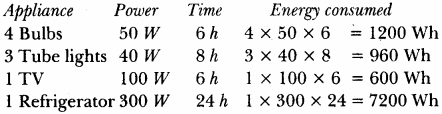Total energy consumed = 9960 Wh = 9.960 kWh
Electricity bill amount = 9.960 units × ₹2.50 = ₹24.90
For 30 days = 30 × 24.90 = ₹747.

Question 3.
(i) Name the physical quantity defined by rate of doing work. Define its SI unit.
(ii) Why is concept of average power useful? How is it determined?
(iii) A boy of mass 45 kg runs up a staircase of 45 steps in 9 s. If the height of each step is 15 cm, find his power.
(g = 10 m/s2)
(i) Power: Watt or J/s. 1 watt is the power of an object which does work at the rate of 1 J per second.

(ii) Power of an object may vary. Hence, average power is important in the case when the average power of the entire process within a given time is calculated.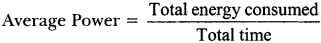(iii) P = $$\frac { mgh }{ t }$$ = $$\frac { (50×10×45×0.15) }{ 9 }$$ = 375 W.

Question 4.
(i) A body of mass 15 kg possesses kinetic energy of 18.75 kJ. Find the velocity.
(ii) An electric bulb of 100 W is used for 4 hours a day. Calculate the energy consumed by it in a day in joules and kilowatt hour unit.
(i) K.E. = $$\frac { 1 }{ 2 }$$ mv2
= 18.75 kJ = 18750
v2 = $$\frac { 18750×2 }{ 15 }$$
v = √2500 m/s = 50 m/s

(ii) E = $$\frac { P }{ t }$$ = 100 W × 4 h = 0.4 kWh
Energy consumed by it in a day = 0.4 × 3.6 × 106 J
= 1.44 × 106 JQuestion 5.
(a) A stone is thrown upwards from a point A, as shown in the figure. After reaching the highest point B, it comes down. Explain the transformation of energy from A to B and B to A and also mention the type of energy possessed by the stone at point A, B and C of its journey.
(b) A body of mass 20 kg is dropped from a height of 100 m. Find its K.E. and P.E. after,
(i) First second
(ii) Second second
(iii) Third second
(a) While moving upward (A to B) K.E → P.E. and while moving downward (B to A)
P.E.→ K.E.
At,
A → K.E.
B → P.E.
C → K.E. + P.E.

(b) Total Energy = mgh
= 20 × 10 × 100 = 2 × 104 J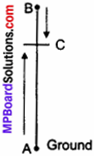(i) After first, second: v = u + gt ms-1
= 10 × 1 = 10 m/s
K.E. = $$\frac { 1 }{ 2 }$$ mv2.
= $$\frac { 1 }{ 2 }$$ × 20 × 10 × 10
= 1000 J
P.E.= T.E. – K.E.
= 20,000 – 1000 = 19,000 J

(ii) After second, second: v = 20 ms-1
K.E. = mgh + $$\frac { 1 }{ 2 }$$ mv2.
= 4,000 J
P.E. = T.E – K.E.
= 20,000 – 4,000 = 16,000 J

(iii) After third, second: v = 30 ms-1
K.E. = mgh + $$\frac { 1 }{ 2 }$$ mv2.
= 9,000 J
P.E. = T.E.- K.E.
= 20,000 – 9,000 = 11,000 J

Work and Energy Higher Order Thinking Skills (HOTS)

Question 1.
Earth and other planets moves continuously around the sun. Do they work?
No, Earth and other planets do not work while moving around the earth because they move in a circular path and reach the initial point after sometimes, so shows no displacement, hence no work is done by earth and other planets.Question 2.
Generally heavy objects exert more power over other objects. Give reason.
As expression, P = wit
On expanding, P = $$\frac { m.a.s }{ t }$$
Here, P is proportional to mass in p × m
Hence, heavy object exert more pressure or power.

VI. Value-Based Question

Question 1.
Ravi saw a lady labour who carried stones on her head from one point of the construction site to the other end which was some 500 m far. He prepares a trolley for the labour to carry the stones, to make her work easier:

1. Is any work done by the labour while carrying the stones from point A to point B on head by lady labour in the construction site?
2. Is any work done by pulling the trolley of stones from point A to point B?
3. What value of Ravi is seen in the above act?